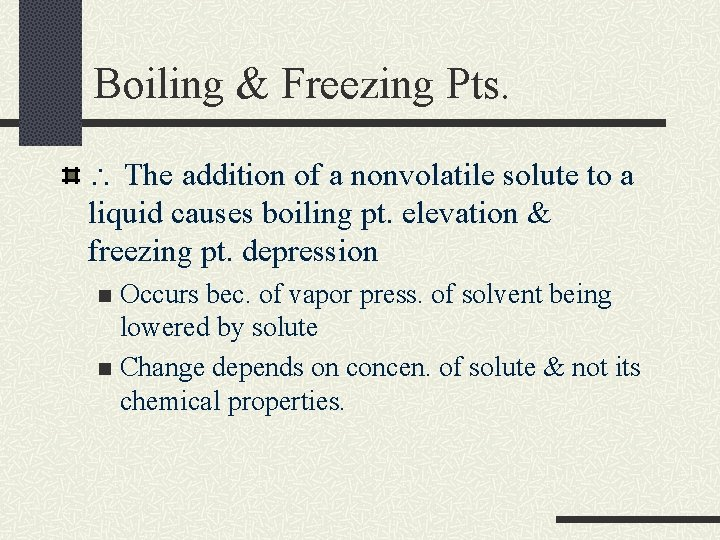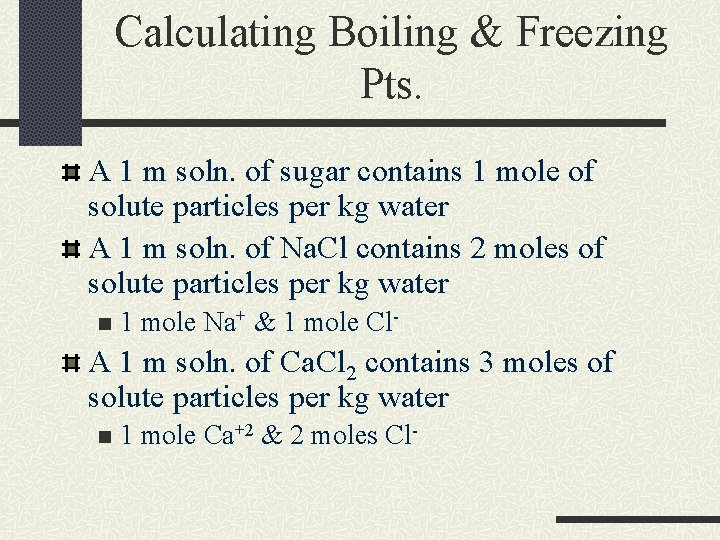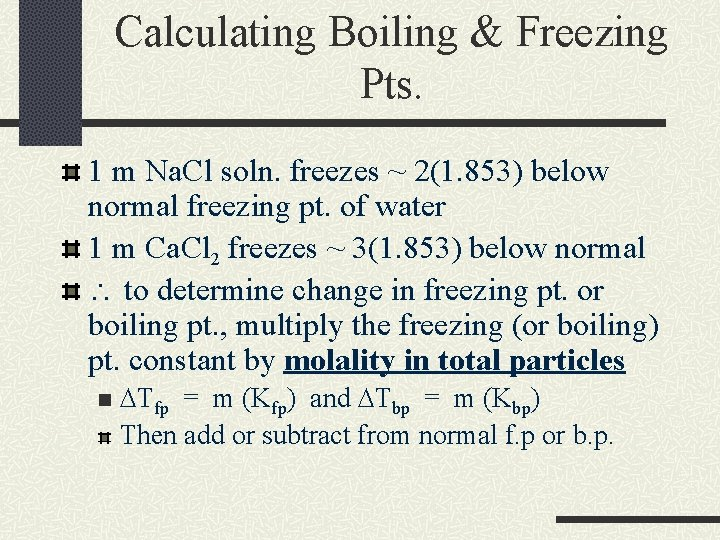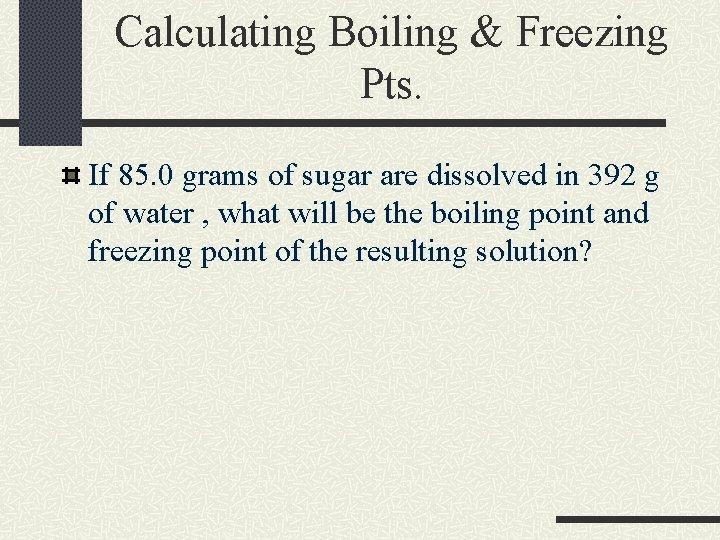# Chapter 21 Boiling and Freezing Points Boiling Freezing

• Slides: 11Chapter 21 Boiling and Freezing PointsBoiling & Freezing Pts. Presence of nonvolatile solute particles causes boiling pt. of soln. to be raised. n Vapor pressure is lowered, need a higher temp. to get vapor press. of liquid to = atmos. press. Freezing Pt. - temp. @ which vapor press of solid & liquid are = n Since addition of solute particles lower vapor press, solns. freeze @ lower temp.Boiling & Freezing Pts. The addition of a nonvolatile solute to a liquid causes boiling pt. elevation & freezing pt. depression Occurs bec. of vapor press. of solvent being lowered by solute n Change depends on concen. of solute & not its chemical properties. nCalculating Boiling & Freezing Pts. 1 mole of nonvolatile solute particles will raise the boiling temp. of 1 kg of water 0. 515 o. C (molal boiling pt. elevation constant for water) The same conc. will lower the freezing pt. of 1 kg of water 1. 853 o. C (molal freezing pt. constant for water)Calculating Boiling & Freezing Pts. A 1 m soln. of sugar contains 1 mole of solute particles per kg water A 1 m soln. of Na. Cl contains 2 moles of solute particles per kg water n 1 mole Na+ & 1 mole Cl- A 1 m soln. of Ca. Cl 2 contains 3 moles of solute particles per kg water n 1 mole Ca+2 & 2 moles Cl-Calculating Boiling & Freezing Pts. 1 m Na. Cl soln. freezes ~ 2(1. 853) below normal freezing pt. of water 1 m Ca. Cl 2 freezes ~ 3(1. 853) below normal to determine change in freezing pt. or boiling pt. , multiply the freezing (or boiling) pt. constant by molality in total particles n D fp = m (Kfp) and D bp = m (Kbp) Then add or subtract from normal f. p or b. p.Calculating Boiling & Freezing Pts. If 85. 0 grams of sugar are dissolved in 392 g of water , what will be the boiling point and freezing point of the resulting solution?Calculating Boiling & Freezing Pts. If 26. 4 g of Nickel (II) bromide are dissolved in 224 g of water, what will be the boiling point and freezing point of the resulting soln. ?Experimental Determination of Molecular Mass Molec. Mass of a solute may be found by using b. p. elevation or f. p. depression if a known mass of solute is added to a known mass of solvent and the resulting shift in b. p. or f. p. is measured.Experimental Determination of Molecular Mass DTbp = m Kbp m = DTbp Kbp Once molality is found, molec. mass (g/mol) can be found mathematically.Experimental Determination of Molecular Mass If 99. 0 g of nonionizing solute are dissolved in 669 g of water, and the freezing point of the resulting soln. is -0. 960 o. C, what it the molecular mass of the solute?